# Parametric Study of the Airflow Structure in a Solar Chimney

Parametric Study of the Airflow Structure in a Solar Chimney

LME Laboratory of Mechanics, Department of Mechanical Engineering. University of Laghouat, Laghouat 03000, Algeria

LABTHOP Laboratory, Faculty of Sciences, University of El Oued, El Oued 39000, Algeria

Kocaeli University, Engineering Faculty, Mechanical Engineering Department, Kocaeli 41001, Turkey

Laboratory of Electromechanical Systems, National School of Engineers of Sfax, University of Sfax, Sfax 3038, Tunisia

Corresponding Author Email:
s.bouabdallah@lagh-univ.dz
Page:
285-292
|
DOI:
https://doi.org/10.18280/ijht.380202
21 March 2020
|
Accepted:
5 June 2020
|
Published:
30 June 2020
| Citation

OPEN ACCESS

Abstract:

Solar chimney converts solar thermal energy into kinetic energy to generate electricity and it is an alternative technology for electricity generation from solar energy. In this work, we are interested in the study of geometrical parameters on convection airflow within a solar chimney. For this, we have considered a two-dimensional prototype model of a solar chimney. The boundary conditions are chosen to be similar to those in reality. The considered mathematical model is a system of partial differential equations formed by the continuity, momentum, and energy equations in conjunction with the standard k-ε turbulence model. The numerical simulations were performed by a commercial Computational Fluid Dynamics (CFD) code based on the finite volume method. Good agreements of the present results with experimental and numerical data were obtained. The effects of the tower and collector radius/ heights on the aerodynamic structure were studied and examined. The obtained results can be used for the design of new solar chimney systems.

Keywords:

convection, solar chimney, geometrical parameters, solar tower, solar collector

1. Introduction

As we have mentioned, solar power has gained particular importance in the current era due to the cleanest source of energy. Also, Solar chimney involves high investment costs and traditionally one presents low efficiency. For this, we are interested in the present work to analyze the behavior of the airflow i.e. velocity and temperature fields in an open cavity applied to a solar chimney. Particularly, we have studied the influence of geometrical dimensions on the efficiency of solar chimney, such as the tower and the collector radius and heights. The remainder of this paper is organized as follows: Section 2 describes the physical problem. Section 3 provides the mathematical formulation and numerical solution process. Section 4 discusses the numerical soltion process, which have been used for the computation, the grid independence study, and the comparison between our predictions and the experimental data results. Section 5 presents the results and discussion: parameteric study of the airflow structure in the solar cheminy such as, tower’s radius and height, collector’s radius and height are presented and discussed. Section 6 contains concluding remarks.

2. Physical Problem

The physical system is a solar chimney prototype used for electricity production (Figure 1). It consists of a tower with a height Ht=12.5 m and a radius Rt=0.5m, mounted on a collector with a radius Rc=12.3m, a height at the entrance Hc2=0.05 m and a height Hc1=0.5m elsewhere. The boundary conditions are imposed to satisfy the air flow conditions through the solar chimney.

We consider some assumptions commonly made in this type of problems, such as: the fluid is Newtonian and incompressible. The large change in the section between the collector and the tower makes it possible to generate strong pressure gradients then high flow velocities which causes the flow regime to be turbulent. Furthmore, the built of the solar chimney has a symmetry axis (in the tower) for this we consider that the flow is axisymmetric. For the fluid, all the thermo-physical properties are constant except the fluid density term, where the volume forces vary linearly with temperature. i.e. the Boussinesq approximation is valid.

## 1.png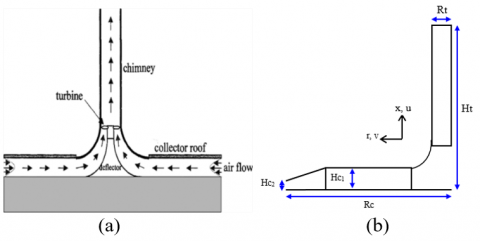Figure 1. A sketch of solar chimney (a), and physical model (b) for the present work

3. Mathematical Formulation

After the introduction of the assumptions given above, we can consider the average Navier-Stokes equations to describe the phenomenon of natural convection airflow inside the solar chimney.

The continuity equation:

$\frac{\partial \rho }{\partial t}+\frac{\partial }{\partial x}\left( \rho u \right)+\frac{1}{r}\frac{\partial }{\partial r}\left( r\rho v \right)=0$  (1)

The momentum equation along the x-direction:

$\frac{\partial }{\partial t}\left( \rho u \right)+\frac{\partial }{\partial x}\left( \rho uu \right)+\frac{1}{r}\frac{\partial }{\partial r}\left( ru\rho v \right)=$

$-\frac{\partial }{\partial x}\left( p+\frac{2}{3}\rho k \right)+\frac{\partial }{\partial x}\left( {{\mu }_{e}}\frac{\partial u}{\partial x} \right)+\frac{1}{r}\frac{\partial }{\partial r}\left( r{{\mu }_{e}}\frac{\partial u}{\partial r} \right)$   (2)

$+\frac{\partial }{\partial x}\left( {{\mu }_{e}}\frac{\partial u}{\partial x} \right)+\frac{1}{r}\frac{\partial }{\partial r}\left( r{{\mu }_{e}}\frac{\partial v}{\partial r} \right)+$$\left(\rho_{0}-\rho\right) g The momentum equation along the r-direction: \frac{\partial }{\partial t}\left( \rho v \right)+\frac{\partial }{\partial x}\left( \rho vu \right)+\frac{1}{r}\frac{\partial }{\partial r}\left( ru\rho v \right)= -\frac{\partial }{\partial x}\left( p+\frac{2}{3}\rho k \right)+\frac{\partial }{\partial x}\left( {{\mu }_{e}}\frac{\partial v}{\partial x} \right)+\frac{1}{r}\frac{\partial }{\partial r}\left( r{{\mu }_{e}}\frac{\partial v}{\partial r} \right) (3) +\frac{\partial }{\partial x}\left( {{\mu }_{e}}\frac{\partial u}{\partial r} \right)+\frac{1}{r}\frac{\partial }{\partial r}\left( r{{\mu }_{e}}\frac{\partial v}{\partial r} \right)-$$2{{\mu }_{e}}\frac{v}{{{r}^{2}}}$

The energy conservation equation:

$\frac{\partial }{\partial t}\left( \rho T \right)+\frac{\partial }{\partial x}\left( \rho uT \right)+\frac{1}{r}\frac{\partial }{\partial r}\left( r\rho vT \right)$

$=\frac{\partial }{\partial x}\left[ \left( \frac{\mu }{\Pr }+\frac{{{\mu }_{t}}}{{{\Pr }_{t}}} \right)\frac{\partial T}{\partial x} \right]+\frac{1}{r}\frac{\partial }{\partial r}\left[ r\left( \frac{\mu }{\Pr }+\frac{{{\mu }_{t}}}{{{\Pr }_{t}}} \right)\frac{\partial T}{\partial r} \right]$  (4)

$+\frac{\beta T}{{{C}_{p}}}\left[ \frac{\partial \rho }{\partial t}+\frac{\partial }{\partial x}\left( \rho u \right)+\frac{1}{r}\frac{\partial }{\partial r}\left( r\rho v \right) \right]$

$+\frac{\beta T}{{{C}_{p}}}\left[ -\rho \frac{\partial u}{\partial x}-\rho \frac{\partial u}{\partial x}-\frac{\rho }{r}\frac{g}{\partial r}\left( rv \right) \right]$

In Eqns. (3)-(4) x, r, u, v, p, t, ρ, r, x, g, β, μ, Pr and T, are the system coordinate, velocity component, pressure, time, volumetric mass, gravity, thermal coefficient of expansion dynamic viscosity, Prandt number and temperature, respectively.

In this study, the turbulent convection regime was handled by the standard k-ε turbulence model, in which, the eddy viscosity μt (Eq. (5)) is described in terms of the turbulent kinetic energy (Eq. (6)) and its dissipation rate ε (Eq. (7)) as follows:

${{\mu }_{t}}=\rho {{C}_{\mu }}\frac{{{k}^{2}}}{\varepsilon }$    (5)

$\frac{\partial }{\partial t}\left( \rho k \right)+\frac{\partial }{\partial x}\left( \rho uk \right)+\frac{1}{r}\frac{\partial }{\partial r}\left( r\rho vk \right)=$$\frac{\partial }{\partial x}\left( \frac{{{\mu }_{e}}}{{{\sigma }_{k}}}\frac{\partial k}{\partial x} \right)+\frac{1}{r}\frac{\partial }{\partial r}\left( r\frac{{{\mu }_{e}}}{{{\sigma }_{k}}}\frac{\partial k}{\partial r} \right)+{{P}_{K}}+{{G}_{K}}-\rho {{C}_{d\varepsilon }}$  (6)

$\frac{\partial }{\partial t}\left( \rho \varepsilon \right)+\frac{\partial }{\partial x}\left( \rho u\varepsilon \right)+\frac{1}{r}\frac{\partial }{\partial r}\left( r\rho v\varepsilon \right)$

$=\frac{\partial }{\partial x}\left( \frac{{{\mu }_{e}}}{{{\sigma }_{\varepsilon }}}\frac{\partial \varepsilon }{\partial x} \right)+\frac{1}{r}\frac{\partial }{\partial r}\left( \frac{{{\mu }_{e}}}{{{\sigma }_{\varepsilon }}}\frac{\partial \varepsilon }{\partial r} \right)$  (7)

$+\left( \frac{{{C}_{1\varepsilon }}}{k}\left( {{P}_{k}}+{{G}_{k}} \right)\left( 1+0.8{{R}_{F}} \right)-{{C}_{2}}\rho \frac{{{\varepsilon }^{2}}}{k} \right)$

In these equations, Pk stands for the source term of the turbulent kinetic energy and is described as:

${{P}_{k}}={{\mu }_{t}}\left[ {{\left( \frac{\partial u}{\partial r}+\frac{\partial v}{\partial x} \right)}^{2}}+2{{\left( \frac{\partial v}{\partial r} \right)}^{2}}+2{{\left( \frac{v}{r} \right)}^{2}} \right]$ (8)

The term Gk represents the production of the turbulent kinetic energy produced by the buoyancy force effects. It is given by:

${{G}_{k}}=-\frac{\beta g}{\rho }\frac{{{\mu }_{t}}}{{{\Pr }_{t}}}\frac{\partial T}{\partial x}$ (9)

The turbulence constants used in the present study are summarized in Table 1.

Table 1. Constants of the standard k-ɛ turbulence model

 Cμ CD C1 C2 σk σɛ 0.09 1 1.44 1.92 1 1.3
4. Numerical Solution Process

The numerical solution of governing equations associated with the boundary conditions was carried out using the finite volume method. The components of the velocity were stored at staggered locations, and the scalar quantities were stored in the centers of these volumes. The Power Law scheme was considered to evaluate the convection terms at the interface of each control volume. The SIMPLER algorithm (Semi Implicit Method Pressure Linked Equation Revised) for coupling pressure-velocity was applied to solve the velocity–pressure coupling. The discretized algebraic equations were solved by the line-by-line tri-diagonal matrix algorithm (TDMA) [28-30].

4.1 Mesh independency test

In this work, we have considered different grid sizes, namely 11×150, 22×250, 32×376 and 42×450 nodes. Figure 3 shows the velocity and temperature distributions near the ground at x = 0.025 m for the different grid sizes. According to these results, the flow parameters values are almost unchangeable when the mesh number is increased to 32×376 and it is fine enough to obtain accurate results. We chose the grid 32×376 better than 22×250, despite being almost coincident for saving the accuracy of the results since comparison with the grid bigger than 42×450 nodes. This grid is chosen for the all simulations along this work for saving computation time without affecting the accuracy of the results (Figure 2).

## 2.jpg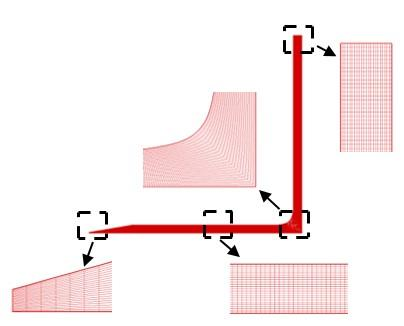Figure 2. Mesh structure of computational domain

4.2 Validation and comparison with anterior results

The obtained numerical results are compared with the experimental and numerical investigations found in the literature. At first, a comparison has been made for the temperature distribution with the experimental results of Maia et al. , as illustrated in Figure 4a. From these results, a slight difference between the data has been observed. This behavior can be caused by the boundary conditions imposed on the collector. A further comparison is made with the velocity distribution presented with the numerical results of Lebbi et al. , as shown in Figure 4b. From these recent results, the obtained results correspond well with those in ref.  corrected by their results.

## 3a.jpg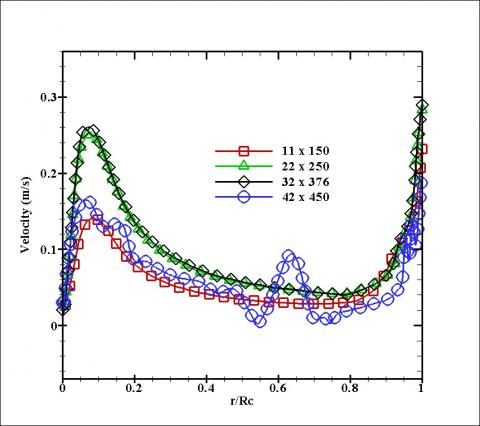(a)

## 3b.jpg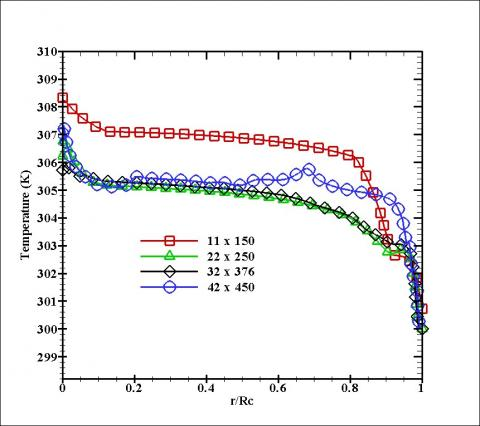(b)

Figure 3. Comparison of velocity profiles (a) and temperature profiles (b) near the ground (at x=0.025m) along the collector radius for various mesh sizes

## 4a.jpg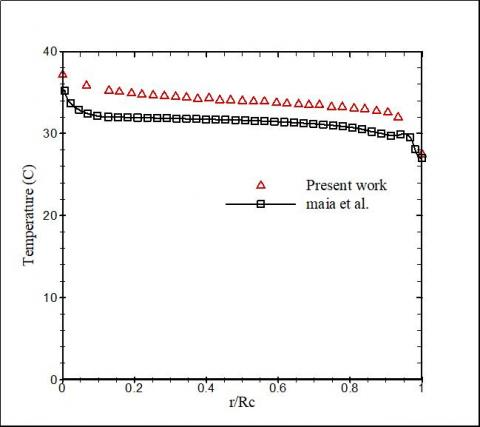(a)

## 4b.jpg(b)

Figure 4. Variation of temperature and velocity profiles vs. collector radius. Comparison of our results with those of; (a) Maia et al.  and (b) Lebbi et al. 

5. Results and Discussions

It is useful to recall that here we consider a solar chimney prototype used for electricity production. An inlet horizontal velocity at ambient temperature (300K) is imposed in the inlet of SC, and it is assumed that boundary conditions of ground velocity are no-slip and imposed temperature is 310K. On the collector, we imposed also the ambient temperature and so-slip velocity condition, and the conditions of the established flow regime of speed and atmospheric pressure at the chimney outlet. Due to the symmetry condition we take only the half of the chimney applying the symmetric conditions at the middle.

5.1 Effect of the tower’s radius (Rt)

In Figure 5, we have presented the velocity and temperature profiles along the collector radius, for three enlargements of tower radius Rt=0.25, 0.5 and 1 m. It is seen from the results presented in the figure that the velocity increases slowly length wise the collector where it takes a maximum value in the interval r/Rc=0.1 to 0.2 which corresponds to the section of the collector-tower junction. The increase in the radius of the tower is directly proportional to the increase of the velocity along the collector, because increasing the section of the tower will create a pressure drop in it, and then causes the air to easily enter the manifold.

## 5a.jpg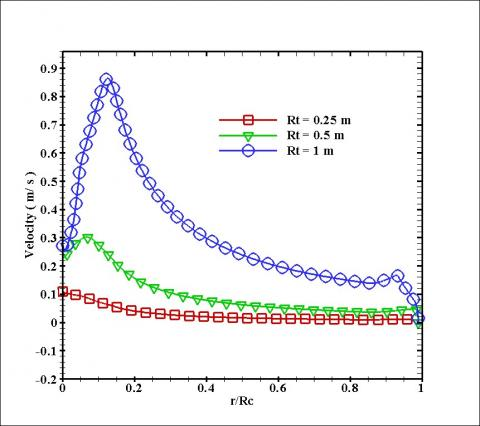(a)

## 5b.jpg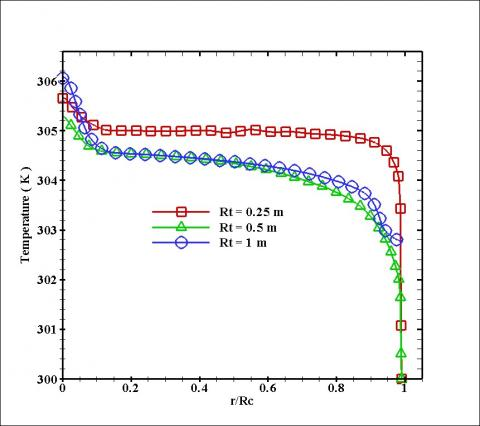(b)

Figure 5. Profiles of the velocity (a) and temperature (b) near the ground (at x = 0.25 m) along the radius of collector, for different radius of tower

For the temperature, an opposite phenomena has been observed since it declines with as the tower radius increases due to the fact that the increment in the radius of the tower leads to an increase in the overall flow of air that passes through the tower. Therefore, during the same period the air is heated in large quantities in the collector, which reduces the flow temperature in the collector.

5.2 Effect of the tower’s height (Ht)

In Figure 6, we have presented the velocity and temperature profiles along the radius of collector, for three tower heights Ht=0.25, 0.5 and 1 m. It is evident from these results that an increment in the tower height increases the air velocity inside the collector. Thus, the higher the flow rate generated by the system, the greater the average velocity values from the collector to the tower. For the profiles of the temperature, it is noticed that the increment of the tower height causes a rise in the temperature in the collector due to the pressure increase when increasing the tower height.

## 6a.jpg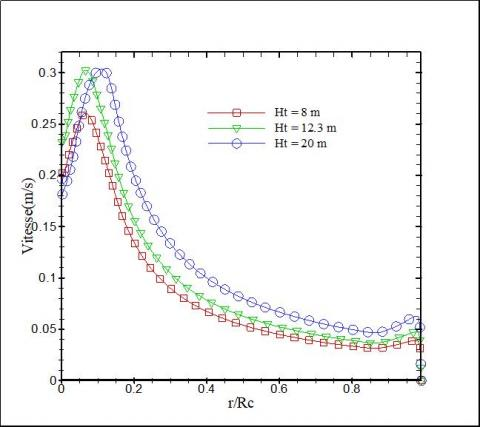(a)

## 6b.jpg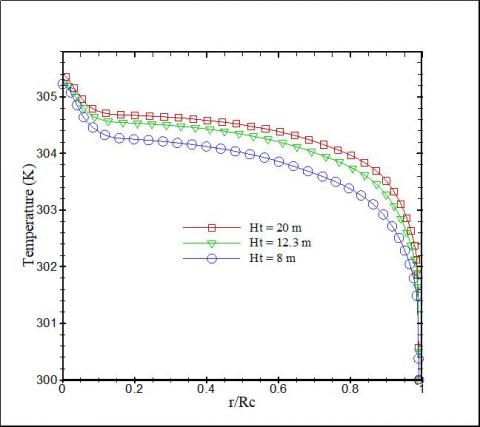(b)

Figure 6. Profiles of the velocity (a) and the temperature (b) near the ground (at x =0.25m) along the radius of collector for different heights of tower

5.3 Effect of the collector’s radius (Rc)

Figure 7 illustrates the profiles of the velocity and temperature in the collector, for four collector radii, equal to Rc=4, 8, 12.5, and 20 m. From these results, it has been noted that at the inlet of the collector which corresponds to r/Rc=1, an increase in cross-flow section has been observed which causes a decrease of the flow rate. This fact is due to the increase in the height of the collector. The temperature changes from the inlet to the outlet of the manifold and increases with increasing radius collector. For collector radius varying from 4 m to 8 m, the air temperature increases. However, for the large radius values at a certain point, the temperature begins to decrease because of the increase of velocity. The interpretation of the increase in temperature at the intersection by reducing the velocity and the adiabatic boundary conditions.

## 7a.jpg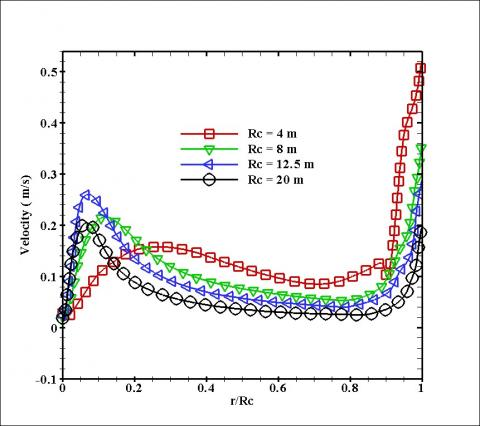(a)

## 7b.jpg(b)

Figure 7. Variation of velocity profiles (a) and temperature profiles (b) in the vicinity of the ground (at x=0.25m) along the radius of collector for different radius of collector

5.4 Effect of the collector’s height (Hc1)

Figure 8 shows the air flow streamlines inside the chimney for four collector height values Hc1=0.25, 0.5, 0.75, and 1m. From these results, it appears that the airflow velocity is higher for the collector with small heights due to reduced flow area. However, the mass-flow rate augments with the increment of the collector height. For cases of Hc1=0.75 and Hc1=1m (Figures 8c-d), the presence of the vortices has been observed in the inlet of collector and the junction section, respectively. The vortices in the center increase in size and move upwards occupying almost the entire chimney. This allows the release of a large amount of heat to the outlet.

## 8a.png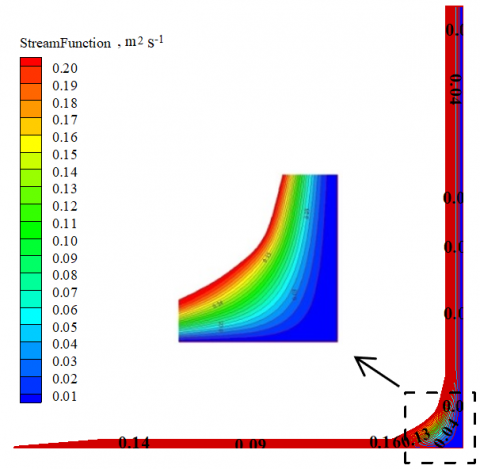(a) Hc1=0.25m

## 8b.png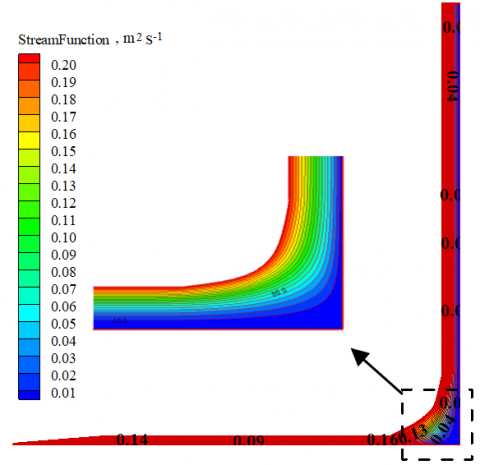(b) Hc1=0.5m

## 8c.png(c) Hc1=0.75 m

## 8d.png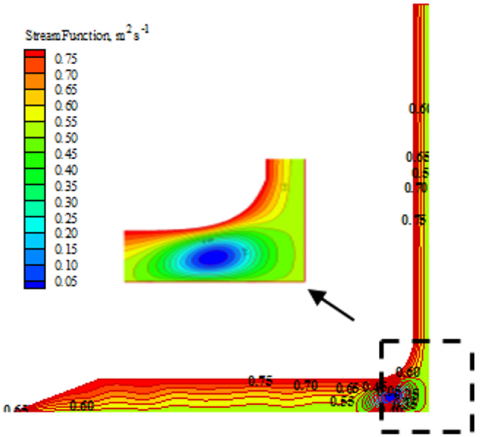(d) Hc1=1m

Figure 8. Streamlines in the SC plotted for different collector heights

6. Conclusion

Since the solar chimney involves high investment costs and traditionally one presents low efficiency, we are interested in the present work to analyze the behavior of the airflow. The effect of the design characteristics on the airflow inside a solar chimney was carried out. The obtained simulation results showed a good agreement with the experimental data found in the literature. Numerical methods presents a good way to optimize performance of the present the solar chimney by considering the geometric factors affecting the power generation. The main obtained results can be summarized as follows:

1. Increasing of the tower’s radius expands the mass airflow through the tower, and increases the productivity in the junction (turbine).

2. An increase in the collector height has a direct impact on the air velocity.

3. An increment in the collector’s radius enhances the thermal exchange from the collector inlet to the outlet and intensifies the flow rate at the junction, hence it increases the kinetic energy transferred to the turbine.

4. The increase of the collector’s height reduces the air velocity near the junction, while the temperature increases as a result of raising the airflow rate which presents a large momentum amounts to rotate the turbine.

5. The gained findings of this study can be helpful for the design and development of the solar chimney systems.

In the future, we propose to develop an experimental model and make it available for other advances.

Acknowledgment

The authors gratefully acknowledge the Directorate General of Scientific Research and Technological Development (DGSRTD) – to support this work, and fund the LME laboratory for carrying out research.

Nomenclature
 g Acceleration of gravitational, m.s-2 k Turbulent kinetic energy, m2.s-2 p Pressure, Pa. Rc Radius of collector, m-1 Rt Radius of tower, m Ht Height of tower, m HC1 Height of collector, m HC2 Collector inlet height, m Tcol Collector temperature, m T0 Ambient temperature, m u, v Components of velocity, m/s r,x Coordinate system, m Greek symbols α Under relaxation factor ρ Density kg.m-3 λ Thermal conductivity, W.m-1.K-1 μ Dynamic Viscosity, kg.m-1.s-1 ε Dissipation rate of the turbulent kinetic energy, m2.s-3 φ Diffusive flux density, W.m-2 Φ Specific Intensity, W.m-2
References

 Maia, C.B., Ferreira, A.G., Vall, R.M., Cortez, F.B.M. (2009). Theoretical evaluation of the influence of geometric parameters and materials on the behavior of the airflow in a solar chimney. Computers and Fluids, 38(3): 625-636. https://doi.org/10.1016/j.compfluid.2008.06.005

 Larbi, S., Bouhdjar, A., Chergui, T. (2010). Performance analysis of a solar chimney power plant in the southwestern region of Algeria. Renewable and Sustainable Energy Reviews, 14(1): 470-477. https://doi.org/10.1016/j.rser.2009.07.031

 Zhou, X.P., Yang, J.K, Xiao, B., Long, F. (2008). Numerical study of solar chimney thermal power system using turbulence model. Journal of the Energy Institute, 81(2): 86-91. https://doi.org/10.1179/174602208X303563

 Ming, T.Z., Liu, W., Xu, G.L., Xiong, Y.B., Pan, Y. (2008). Numerical simulation of the solar chimney power plant systems coupled with turbine. Renewable Energy, 33(5): 897-905. https://doi.org/10.1016/j.renene.2007.06.021

 Chergui, T., Larbi, S., Bouhdjar, A. (2010). Thermo-hydrodynamic aspect analysis of flows in solar chimney power plants-a case study. Renewable and Sustainable Energy Reviews, 14(5): 1410-1418. https://doi.org/10.1016/j.rser.2010.01.017

 Chergui, T., Larbi, S., Bouhdjar, A. (2011). Configuration aspect analysis in solar chimney power plants using finite element method. Procedding of the Eleventh World Renewable Energy Congress, WREC’2011, Linköping, Sweden.

 Bernardes, M.A.S., Valle, R.M., Cortez, M.F.B. (1999). Numerical analysis of natural laminar convection in a radial solar heater. International Journal of Thermal Science, 38(1): 42-50. https://doi.org/10.1016/S0035-3159(99)80015-4

 Patel, S.K., Prasad, D., Rafiuddin, A.M. (2014). Computational studies on the effect of geometric parameters on the performance of a solar chimney power plant. Energy conversion and Management, 77: 424-431. http://dx.doi.org/10.1016/j.enconman.2013.09.056

 Guo, P., Li, J., Wang, Y., Wang, Y. (2015). Numerical study on the performance of a solar chimney power plant. Energy Conversion and Management, 105: 197-205. https://doi.org/10.1016/j.enconman.2015.07.072

 Amirkhani, S., Nasirivatan, Sh., Kasaeian, A.B., Hajinezhad, A. (2015). ANN and ANFIS models to predict the performance of solar chimney power plants. Renew Energy, 83: 597-607. https://doi.org/10.1016/j.renene.2015.04.072

 Roozbeh, S., Majid, A., Behzad, H. (2011). Modeling and numerical simulation of solar chimney power plants. Solar Energy, 85(5): 829-838. https://doi.org/10.1016/j.solener.2011.01.011

 Ghalamchi, M., Kasaeian, A., Ghalamchi, M. (2015). Experimental study of geometrical and climate effects on the performance of a small solar chimney. Renewable and Sustainable Energy Reviews, 43: 425-431. https://doi.org/10.1016/j.rser.2014.11.068

 Nasirivatan, S., Kasaeian, A., Ghalamchi, M., Ghalamchi, M. (2015). Performance optimization of solar chimney power plant using electric/corona wind. Journal of Electrostatics, 78: 22-30. https://doi.org/10.1016/j.elstat.2015.09.007

 Koonsrisuk, A. (2013). Comparison of conventional solar chimney power plants and sloped solar chimney power plants using second law analysis. Solar Energy, 98(Part A): 78-84. https://doi.org/10.1016/j.solener.2013.02.037

 Zou, Z., He, S. (2015). Modeling and characteristics analysis of hybrid cooling-tower-solar-chimney system. Energy Convers Manage, 95: 59-68. https://doi.org/10.1016/j.enconman.2015.01.085

 Bernardes, M.A., Zhou, X. (2013). Strategies for solar updraft tower power plants control subject to adverse solar radiance conditions. Solar Energy, 98: 34-41. https://doi.org/10.1016/j.solener.2013.06.007

 Lebbi, M., Chergui, T., Boualit, H., Boutina, I. (2014). Influence of geometric parameters on the hydrodynamics control of solar chimney. International Journal of Hydrogen Energy, 39(27): 15246-15255. https://doi.org/10.1016/j.ijhydene.2014.04.215

 Jemli, M.R., Naili, N., Farhat, A., Guizani, A. (2016). Experimental investigation of solar tower with chimney effect installed in CRTEn, Tunisia. International Journal of Hydrogen Energy, 41(13): 1-11. https://doi.org/10.1016/j.ijhydene.2016.07.044

 Jianlan, L., Hongjing, G., Shuhong, H. (2016). Power generation quality analysis and geometric optimization for solar chimney power plants. Solar Energy, 139: 228-237. https://doi.org/10.1016/j.solener.2016.09.033

 Koonsrisuk, A., Lorente, S., Bejan, A. (2010). Constructal solar chimney conﬁguration. International Journal of Heat and Mass Transfer, 53(1-3): 327-333. https://doi.org/10.1016/j.ijheatmasstransfer.2009.09.026

 Bernardes, M.A., Von Backstrom, T.W. (2010). Evaluation of operational control strategies applicable to solar chimney power plants. Solar Energy, 84(2): 277-288. https://doi.org/10.1016/j.solener.2009.11.009

 Bernardes, M.A., Zhou, X. (2013). On the heat storage in Solar Updraft Tower collectors Water bags. Solar Energy, 91: 22-31. https://doi.org/10.1016/j.solener.2012.11.025

 Bernardes, M.A., Weinrebe, G. (2003). Thermal and technical analyses of solar chimneys. Solar Energy, 75(6): 511-524. https://doi.org/10.1016/j.solener.2003.09.012

 Al Touma, A., Ghali, K., Ghaddar, N., Ismail, N. (2016). Solar chimney integrated with passive evaporative cooler applied on glazing surfaces. Energy, 115(Part 1): 169-179. https://doi.org/10.1016/j.energy.2016.09.020

 Ghalamchi, M., Kasaeian, A., Ghalamchi, M., Mirzahosseini, A.H. (2016). An experimental study on the thermal performance of a solar chimney with different dimensional parameters. Renewable Energy, 91: 477-483. https://doi.org/10.1016/j.renene.2016.01.091

 Nizetic, S., Penga, Z., Arıcı, M. (2017). Contribution to the research of an alternative energy concept for carbon free electricity production: Concept of solar power plant with short diffuser. Energy Conversion and Management, 148: 533-553. http://dx.doi.org/10.1016/j.enconman.2017.05.062

 Penga, Z., Nizetic, S., Arıcı, M. (2019). Solar plant with short diffuser concept: Further improvement of numerical model by included influence of guide vane topology on shape and stability of gravitational vortex. Journal of Cleaner Production, 212: 353-361. https://doi.org/10.1016/j.jclepro.2018.12.021

 Ghernaout, B., Ghernaout, D., Bouabdallah, S. (2017). Two transitions of thermosolutal natural convection in the presence of an external magnetic field. Mathematical Modelling of Engineering Problems, 4(3): 120-125. http://doi.org/10.18280/mmep.040302

 Ghernaout, B., Attia, M.E.H., Bouabdallah, S., Driss Z., Benali, M.L. (2020). Heat and fluid flow in an agricultural greenhouse. International Journal of Heat and Technology, 38(1): 92-98. https://doi.org/10.18280/ijht.380110

 Bouabdallah, S., Bessaïh, R., Ghernaout, B., Benchatti, A. (2011). Effect of an external magnetic field on the 3-D oscillatory natural convection of molten gallium during phase change. Numerical Heat Transfer; Part A: Applications, 60(1): 84-105. https://doi.org/10.1080/10407782.2011.588558